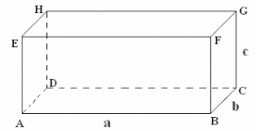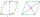# Base side

In a quadrilateral prism, are known surface area S = 12400 mm2 base side m = 40mm and prism height = 120mm, what is the length of base side n =?

n =  8.75 mm

### Step-by-step explanation:Did you find an error or inaccuracy? Feel free to write us. Thank you!#### You need to know the following knowledge to solve this word math problem:

We encourage you to watch this tutorial video on this math problem:

## Related math problems and questions:Calculate the surface of a quadrilateral prism according to the input: Area of the diamond base S1 = 2.8 m2, length of the base edge a = 14 dm, height of the prism 1,500 mm.
• The ABCDThe ABCD trapezoid has a base length of a = 120mm, c = 86mm and an area of S = 2575 mm2. Calculate the height of the trapezoid.
• A trapezoid 3A trapezoid ABCD has the bases length of a = 120 mm, c = 86 mm and the area A = 2,575 mm2. Find the height of the trapezoid.
• Four prismsQuestion No. 1: The prism has the dimensions a = 2.5 cm, b = 100 mm, c = 12 cm. What is its volume? a) 3000 cm2 b) 300 cm2 c) 3000 cm3 d) 300 cm3 Question No.2: The prism base is a rhombus with a side length of 30 cm and a height of 27 cm. The height of t
• TerezaThe cube has an area of base 256 mm2. Calculate the edge length, volume, and area of its surface.
• Sphere A2VThe surface of the sphere is 241 mm2. What is its volume?The surface of the regular quadrilateral prism is 8800 cm2, and the base edge is 20 cm long. Calculate the volume of the prism
• Edge of prismThe regular quadrilateral prism has a surface of 250 dm2, its shell has a content of 200 dm2. Calculate its leading edge.
• Regular prismThe regular four-sided prism has a base of 25 cm2 and a surface of 210 cm2. Find its volume.
• The cylinder baseThe cylinder with a base of 8 dm2 has a volume of 120 liters. From a cylinder filled with water, 40 liters of water was removed. At what height from the bottom /with precision to dm/ is the water level?
• Six paintersSix painters paint an area of 120 m2 in 4 hours. How long does it take for 12 painters to paint 480 m2 with the same performance?
• The cubeThe cube has a surface area of 216 dm2. Calculate: a) the content of one wall, b) edge length, c) cube volume.
• The tankThe tank is full up to 4/5 of the total height and contains 240 hl of water. The area of the base is 6 square meters. What is the height of the tank?
• CylinderThe cylinder surface is 922 dm2. Its height is equal to the radius of the base. Calculate the height of this cylinder.
• The areaThe area of a rhombus is 143 m2. If the longer diagonal is 26 m, find the shorter diagonal in inches named d1.
• Cube wallThe surface of the first cube wall is 64 m2. The second cube area is 40% of the surface of the first cube. Determine the length of the edge of the second cube (x).
• Edge cFind the edge c of cuboid if an edge a = 20 mm, b = 30 mm and surface area S = 8000 mm2.